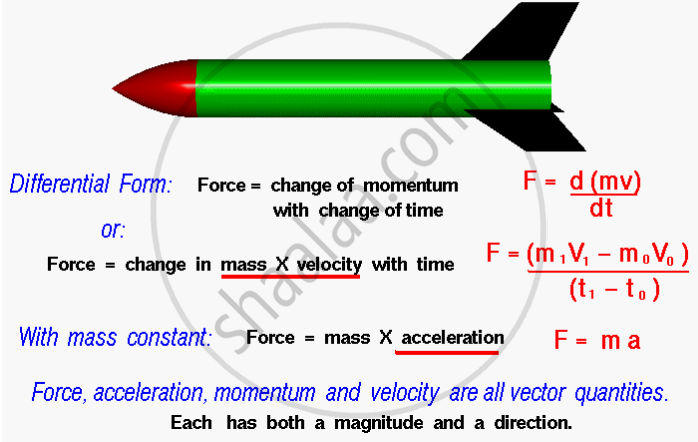Advertisement Remove all ads

# Newton's Second Law of Motion

Advertisement Remove all ads

### Second Law of Motion

“The rate of change of momentum of an object is proportional to the applied unbalanced force in the direction of the force.”This law of motion is also called the Law of Force and Acceleration. It means that force is equal to the change in momentum per change in time. For a constant mass, force equals mass times acceleration.

#### notes

MATHEMATICAL FORMULATION OF SECOND LAW OF MOTION

Suppose an object of mass, m is moving along a straight line with an initial velocity, u. It is uniformly accelerated to velocity, v in time, t by the application of a constant force, F throughout the time, t. The initial and final momentum of the object will be, p1 = mu and p2 = mv respectively.

The change in momentum
∝ p2 – p1
∝ mv – mu
∝ m × (v – u).

The rate of change of momentum ∝ "m" xx "(v - u)"/"t"

or, the applied force,

"F" ∝ "m"xx"(v - u)" / "t"

"F" ∝ "km"xx"(v - u)" / "t"

=kma

Here a = [(v – u)/t] is the acceleration, which is the rate of change of velocity. The quantity, k is a constant of proportionality.

The SI units of mass and acceleration are kg and m/s2 respectively.

The unit of force is so chosen that the value of the constant, k becomes one. For this, one unit of force is defined as the amount that produces an acceleration of 1 m/s2 in an object of 1 kg mass.

That is,

1 unit of force = k × (1 kg) × (1 m/s2).

Thus, the value of k becomes 1.

F = ma

The unit of force is kg m s-2 or newton, which has the symbol N. The second law of motion gives us a method to measure the force acting on an object as a product of its mass and acceleration.

If you would like to contribute notes or other learning material, please submit them using the button below.

#### Video Tutorials

We have provided more than 1 series of video tutorials for some topics to help you get a better understanding of the topic.

Series 1

Series 2

### Shaalaa.com

Newton's 2nd second law, Momentum [00:21:02]
S
##### Series: series 1
0%

Advertisement Remove all ads
Share
Notifications

View all notifications

Forgot password?
Course